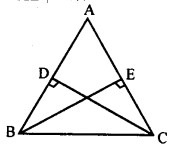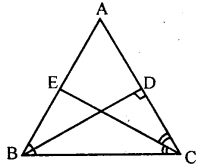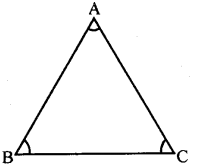# KSEEB Solutions for Class 8 Maths Chapter 11 Congruency of Triangles Ex 11.4

Students can Download Maths Chapter 11 Congruency of Triangles Ex 11.4 Questions and Answers, Notes Pdf, KSEEB Solutions for Class 8 Maths helps you to revise the complete Karnataka State Board Syllabus and score more marks in your examinations.

## Karnataka Board Class 8 Maths Chapter 11 Congruency of Triangles Ex 11.4

Question 1.
In the given figure. If AB || DC and P is the midpoint of BD prove that P is also the midpoint of BD prove that p is also the midpoint of AC.In ∆ PDC and ∆ PAB
PD = PB (data)
∠DPC = ∠APB [V.O.A]
∠PD∆ = ∠PBA [Alternate angles AB || CD]
∴ ∆ PDC ≅ ∆ PAB [ASA postulate]
∴ PC = PA [Corresponding sides]
∴ P is the midpoint AC

Question 2.
In the adjacent figure, CD and BE are altitudes of an isosceles triangle ABC with AC = AB prove that AE = AD.AC = AB [data]
∠ADC = ∠AEB[BE & CD altitudes]
∠DAC =∠EAB [Common angle]

Question 3.
In the figure, AP and BQ are perpendiculars to the line segment AB and AP = BQ. Prove that O is the midpoint of line segment AB as well as PQ.In ∆APO and ∆BQO
AP = BQ [data]
∠POA = ∠BOQ [ V.O.A]
∠PAO = ∠QBO = 90°
[AP & BQ are perpendiculars]
∴ ∆APO = ∆BQO [AS∆ postulate]
∴ AO = BO [Corresponding sides]
PO = OQ
∴ O is the midpoint of AB and PQ.

Question 4.
Suppose ABC is an isosceles triangle with AB = AC, BD and CE are bisectors of|fi andlC. Prove that BD = CE.Ans.
In ∆ABD and ∆ACE
AB = AC [data]
∠ABD = ∠ACE [∠B =∠C and BD and CE are anglar bisectors]
∴ ∆ ABD ≅ ∆ ACE [AS∆ postulate]
BD = CE [Corresponding sides]

Question 5.
Suppose ABC is an equiangular triangle. Prove that it is equilateral. (You have seen earlier that an equilateral triangle is equiangular. Thus for triangles equiangularity is equivalent is equilaterality.